## 墨卡托投影转换详解

263次阅读

X轴：由于赤道半径为6378137米，则赤道周长为2*PI*r = 20037508.3427892，因此X轴的取值范围：[-20037508.3427892,20037508.3427892]。

`2 * Math.PI * 6378137 = 40075016.0019724`

X轴取值范围确实是[-20037508.3427892,20037508.3427892]，但是请不要闭着眼睛说这是周长（实际是半周长）P的经纬度为都为弧度），相邻点Q的经纬度为，那么: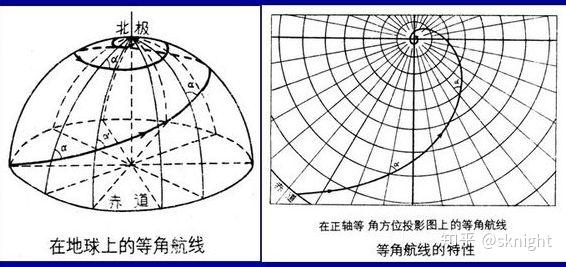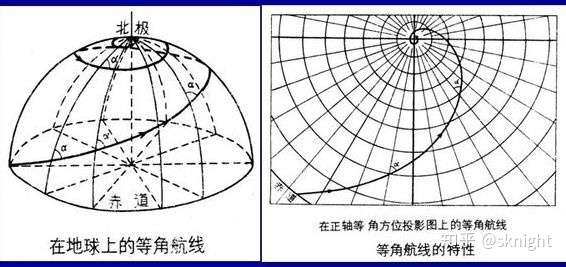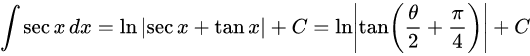的原函数为的反函数称为高德曼函数,（这里由于为了和截图对应需要把变为了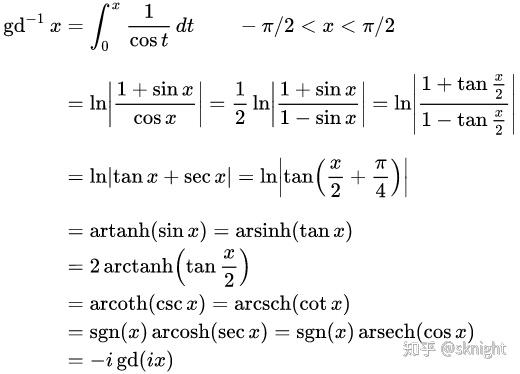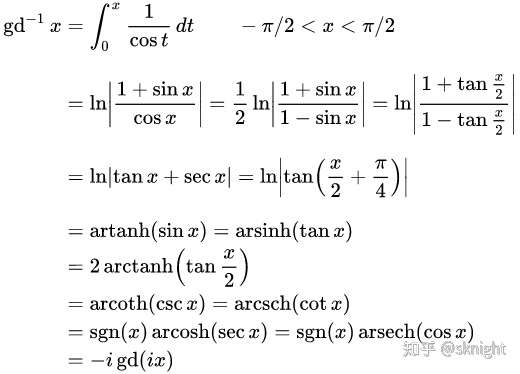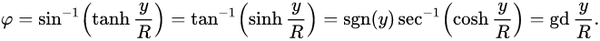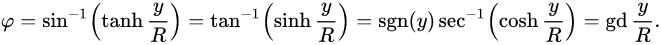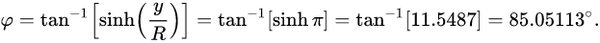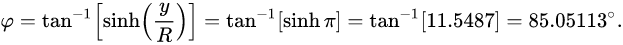```// 弧度版
const x = R * lng
const y = R * Math.log(Math.tan((Math.PI*0.25) + (0.5 * lat)))

// 角度版
const radians = Math.PI / 180
const x = R * lng * radians
const y = R * Math.log(Math.tan((Math.PI*0.25) + (0.5 * lat * radians)))```